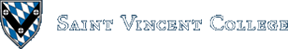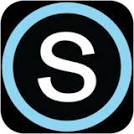Computing & Information Systems    DepartmentSearch CIS Site      Tutorials

Software Design Using C++

Input, Output, and Simple Computations

Output and a First C++ Program

One of the most basic things that you can do in a program is to write some output to the screen. In C++ this is usually done with `cout` as in the following example:

 `````` cout << "This is the message that would appear on screen." << endl; ``````

Note that a message string is enclosed in double quotes. The `<<` symbols point in the direction of data flow: from the message string to the `cout` output stream. The `endl` moves the cursor to the beginning of the next line on the screen. Finally, note that the command, like all C++ statements, ends with a semicolon.

So, how do you incorporate an output statement like the above into a C++ program? Read the output.cpp example, which is copied here for convenience:

 `````` /* Filename: output.cpp Author: Br. David Carlson Date: January 4, 2000 Modified: June 26, 2000 This program merely prints some messages on the screen. */ #include using namespace std; int main(void) { cout << "Start of program" << endl; cout << endl << "You can print whatever you like!" << endl; cout << endl << "End of program" << endl; return 0; } ``````

Items enclosed by /* and */ are comments and are ignored by the compiler. The above example shows the type of comments expected at the top of each program. This section should give the filename, author, date, and a brief (but complete) description. The description should explain what the program does overall, its inputs, and its outputs.

A short comment that fits on one line can be started with the // symbols. Anything on the same line but coming after the // is taken as a comment and is ignored by the compiler. Consider the following two examples:

 `````` // This is a comment. cout << "Hello" << endl; // This is a comment, too. ``````

Let's return to the sample program that we were discussing. The `iostream` header is included whenever you want to do input or output. Since most programs use input and output, you will nearly always want `iostream`. Note that older C++ compilers have a similar `iostream.h` header that can be included. Some compilers support both. In our examples we will use the version of the header without the .h extension. (Warning: sometimes the two versions do not give exactly the same capabilities.) When using headers without the .h extension, also use the `std` namespace as shown. This namespace is needed in order to have access to many items that we will use in our programs.

Sometimes we will need to include other headers in order to use a certain type or class (such as `fstream`) or to be able to use a specialized function (such as sqrt). If you want to use a certain specialized function supplied by the compiler, the compiler's online help should tell you what header(s) to include. A good reference book is also helpful on this.

The program above consists of just a main function. All C++ programs must have a main function. They can also have other functions. program begins at the top of the main function. There are other ways to write a main function (such as using `void`, not `int`, in front of `main`, but the above method is about very common and will be used throughout these notes. The `int` in front of `main` indicates that the main function will return an integer value to the operating system when the function is finished. You can see that the last line of the main function does indeed return the value 0. (Especially in the UNIX operating system, this return value can be used to check on how a program finished. For example, 0 could be used for successful termination and some other number could be returned to indicate an error condition. We will not really use `main`'s return value in our examples.)

Execution of the program begins at the top of the main function. Note that the commands to be carried out by the main function are listed in sequence inside of curly braces. These commands are carried out in sequence, one after the other, unless they contain something that alters this standard order of execution. The last command inside of the main function is typically `return 0`.

Note that the commands inside of a function (and the braces) are indented a uniform 3 spaces. This is a typical indenting style. Although the compiler does not care about indenting, it makes it much easier for those people who must read the program. In this case, it makes it clearer what statements belong to the main function.

See proto.cpp for an outline of a typical C++ program file. It shows how the comment section should be laid out, where to include the headers, etc.

Compiling and Running a Program

Now that we have a C++ program, what do we do with it? Before we can run it on a computer, the program has to be compiled (translated into machine code). Then you run the program itself. Exactly how to do all of this depends very much on what compiler you are using. Read Compiler Considerations, especially the section on Using Microsoft Visual C++, if this is your compiler. Note that if you already have a C++ source code file (such as the `output.cpp` above), you can get a quick start by just double clicking on the filename in MyComputer and accepting the suggestion of building a default workspace. If you are creating a new program from scratch it is better to follow the directions in Compiler Considerations.

Input of Integer, Float, and Character Data

If we can do output, surely we can also do input. The usual method involves reading data from the `cin` input stream. This normally reads its data from what the user types at the keyboard. For example, to read in an integer (`int` as it is called), you might use:

 `````` int num; cin >> num; ``````

Note the use of the variable `num`. A variable is used to hold a value, much like a variable in algebra. In C++ all variables must be declared. Here the variable `num` is declared to be of type `int`, which means that it can hold a whole number (within some reasonable range). A variable is implemented as a named memory location. Thus, the name `num` refers to some location in the computer's main memory where the value for `num` is stored. Our input statement is one way in which to place a value into the variable `num`. Note that the >> symbols point in the direction of data flow: from the `cin` stream to the variable.

Look at iotest.cpp for an example program that uses both input and output. This program takes the common approach of printing a prompt before each input operation. That way, the user of the program is instructed about what to enter at each point. Note the use of two new data types: `char` and `float`. A `char` variable can hold one single character (letter, digit, or special symbol such as +). A `float` holds a real value (which can be a whole number or number with a decimal point, such as 3.1416). Once again there is a certain range of legal values for a `float`, but this range is large enough that we can put off worrying about it for now.

Assignment Statements

Can we do something other than input and output? One thing we can do is to assign a value into a variable. This is a second way to place a value into a variable. The simplest form of the assignment statement is as seen in the following example:

 `````` int num; num = 45; ``````

A single = sign is used for assignment. It does not mean "equals" as in algebra. (C++ uses ==, two equals signs, for equals.) The = means assignment. An assignment statement is always executed from right to left. First, the value on the right hard side is figured out (here the literal number 45) and is then copied into the variable on the left hand side (here the variable `num`). Thus, our example copies 45 into the variable.

The left hand side of an assignment statement needs to be a variable, something that can be assigned a value. The right hand side can be an expression. An expression is anything that evaluates to a reasonable value. For example, if we have an integer variable on the left hand side of the = sign, we could use anything that has an integer value on the right hand side. Here are some examples using integer variable and values:

 `````` int num, answer, temp, value; num = 45; answer = num + 1; temp = num; value = 2 * num + answer; ``````

As before, we place 45 into `num`. The second assignment statement first figures out the value of its right hand side as 46 and then copies the 46 into answer. The next one copies 45 from `num` into `temp`. The last one looks up the values of `num` and `temp` to figure out its right hand side value (as 136) and then copies the 136 into `value`.

Assignment statements can also be used to put character data into character variables. A literal character value must be written with single quotes around it, as in 'A', whereas a literal integer or floating point value is written without quotes, as in 3.1416. Here is an example using some assignment statements with character variables:

 `````` char Ch, Save; Ch = 'T'; Save = Ch; ``````

In this short example, the character T is copied into variable `Ch`. The second assignment statement starts on the right hand side, evaluating `Ch` as 'T'. This character is then copied into the variable `Save`.

Simple Computations

Assignment statements can be used to carry out simple computations. At last we can compute something! For example, to find the area of a rectangle we would want to multiply the length by the width and probably then store the answer in some variable. The computation could be carried out by an assignment statement such as:

 `````` Area = Length * Width; ``````

Look at area1.cpp for an example program that finds the area of a rectangle. It uses `float` variables since the length, width, and area might not be whole numbers. Note how the user is prompted to enter the length and width. Note, too, how different sections of the program are separated by blank lines. That is not too important in such a short program, but in longer ones in can help greatly in improving readability. Consistently indenting by 3 spaces all of the items inside of the function is also used as before.

Back to the main page for Software Design Using C++

Author: Br. David Carlson with contributions by Br. Isidore Minerd
Last updated: August 27, 2009
Disclaimer